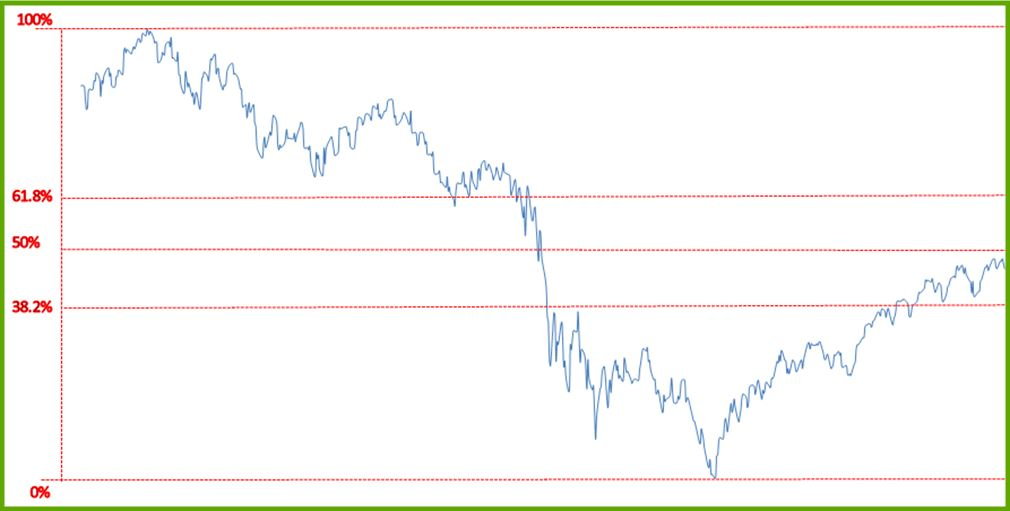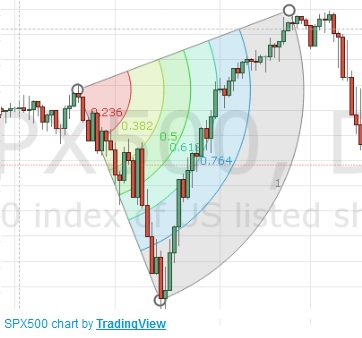# Fibonacci Arcs and Fibonacci Retracement

## Technical Analysis

Fibonacci Arcs and retracements are used as a technical indicator to determine support and resistance. As with most indicators it can be used to see if a breakout has occurred or if a reversal is likely to happen.

## Fibonacci Number

To understand the Fibonacci arcs and retracements, we must first understand where the Fibonacci numbers comes from. The Fibonacci numbers are found by starting with 1,1.  The next number in the sequence is found by adding the previous two numbers. To see it in action, consider this sequence of Fibonacci Numbers:

1,1,2,3,5,8,13,21,34,55,89,144,233,377,610,987,1597

To get them, we go like this:

• 1 = 2
• 1= 3
• 2 + 3 = 5
• 3 + 5 = 8
• 5 + 8 = 13
• 8 + 13 = 21
• 13 + 21 = 34
• 21 + 34 = 55
• 34 + 55 = 89
• 55 + 89 = 144
• 89 + 144 = 233
• 144 + 233 = 377
• 233 + 377 = 610
• 377 + 610 = 987
• 610 + 987 = 1597

We can use these numbers to determine ratios. As the numbers in the sequence get larger, the ratio between them converges to a constant. These ratios for the basis for the Fibonacci arcs and retracements.

By dividing a number by the number after it (ex:610/987) we will get 0.618.
By dividing the number by two after it (ex:610/1957) you get 0.382.
By dividing a number by the number before it we get 1.618.
Note:Other numbers can also be found by varying the numbers we use in the sequence.

These numbers are extremely important not just in finance, but in nature as well and can be seen nearly everywhere. (1.618 and 0.618 is termed the “Golden Ratio” because 1/1.618 = 0.618 among other things). In statistics, the percentage of a sample population found in a normal distribution in within half a standard deviation is 38.2% (which is what you get when you divide a Fibonacci number by two before it). The sheer amount of times we see these numbers in nature and elsewhere is enough to give some credit as to the use of the Fibonacci sequence.

## Fibonacci Retracement

This tool, though less useful than the Fibonacci arc, can be used to determine likely support and resistance levels and can help with past chart patterns as well.
Drawing lines at the high a low, and at 38.2% 50% and 61.8% will provide important support and resistance.

As we can see below, though useful, the retracement only provides so much information and is generally less useful than drawing your own support and resistance lines.What’s important here is that you can see is on the way down the stock went through three lines very quickly but stayed in the top and bottom tiers for quite a long time.

## Fibonacci Arc

Fibonacci Arcs are far more useful as they take into account the time as well as the price.From this graph we can see that the arc follows the 61.8% line very closely before it cuts into the next percentage area. This is very predictable since it couldn’t possibly continue to rise at the rate it was and was a support arc for that period. As with most technical indicators, Fibonacci Arcs and retracements are a tool among many others. They may provide lots of information in one graph and very little in another. What’s important to note is that it would very unlikely for the trades to go from the 100% arc to the 50% arc without some time passing by.